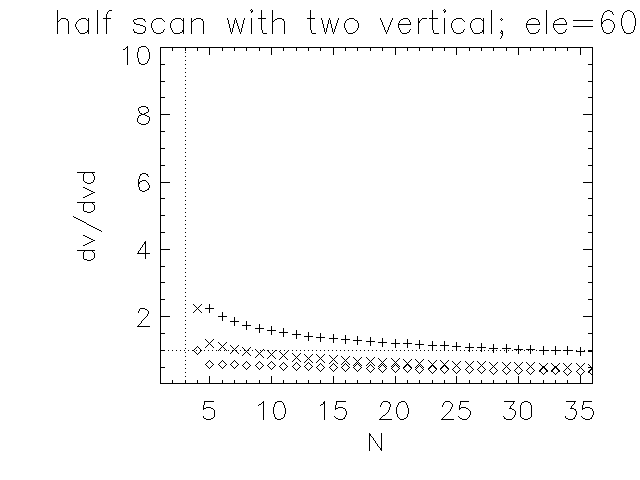# Wind profiler errors

Error estimates for different scan patterns of a Doppler wind profiler as a function of the number of beams.
The error estimate considers only the uncertainty of the Doppler retrieval but not the error due to the separation of the beams and turbulence.
Three principle scan patterns are considered:

• azimuth scans where N beams are distributed evenly between 0 and 360deg
• half scans where the beams are distributed over a half circle from 0 to 180deg
• sector scans where the beams are distributed over one sector from 0 to 90deg
These scans are then expanded by one or two vertical beams.
Errors Δv=dv are given as mutliples of the basic error of the doppler retrieval Δvd=dvd.
dvd is typically in the order of a few cm/s.
Elevation of the tilted beams is allways 75deg.
+ symbols denote the error for the u and v component,
⋄ symbols the error estimate for vertical velocity.
× symbols denote the error for the v component (only for half scans),

## summary

• The lower the elavation / the larger zenith dstance the lower the error.
• Errors of the sector scan are larger than those of the half scan which are larger then tose of the (full) azi scan.
• The classical three beam swinging scan (3DBS) with one vertical beam and two tilted in eastern and western direction can be found in the sector scan with one vertical beam at N=3.
Its errors are dv/dvd=5.4 and dw/dvd=1
• Interestingly an azimuth scan with just three tilted beams (3TB) at 0, 120 and 240deg azimuth has much smaller errors in all three components then the 3DBS:
dv/dvd=3.4 and dw/dvd=0.6
• Adding one or two vertical beams decreases the error in w only slightly. The error in u and v is not affected.
• A sector scan with no vertical beam is awful bad: errors are very large.
• A sector scan with one vertical beam and a tilted beam in norhteastern direction (N=4) has smaller error in the horizontal than 3DBS but is not much better in the vertical:
dv/dvd=4.5 and dw/dvd=0.9
• Adding one addtional vertical beam decreases dw but also dv:
dv/dvd=4.0 and dw/dvd=0.8
• Increasing the number of tilted beams by one such that we use 0, 30, 60, 90deg azimuth (->N=6) decrease dv further:
dv/dvd=3.8 and dw/dvd=0.8
• But all these sector scans still have larger errors than the 3TB scan.

## plots

scan type ele=75deg ele=60deg
full azi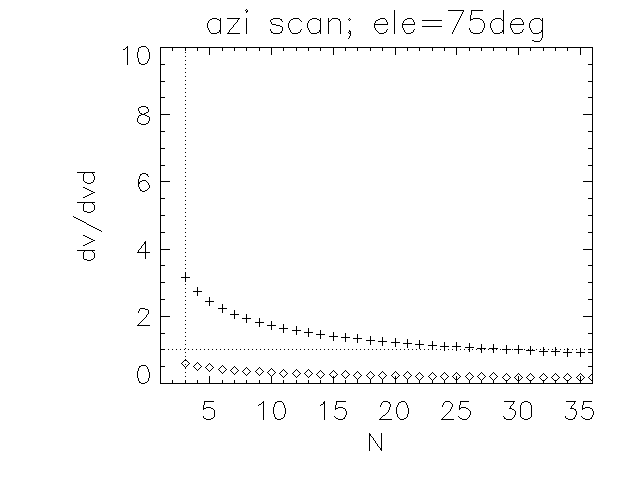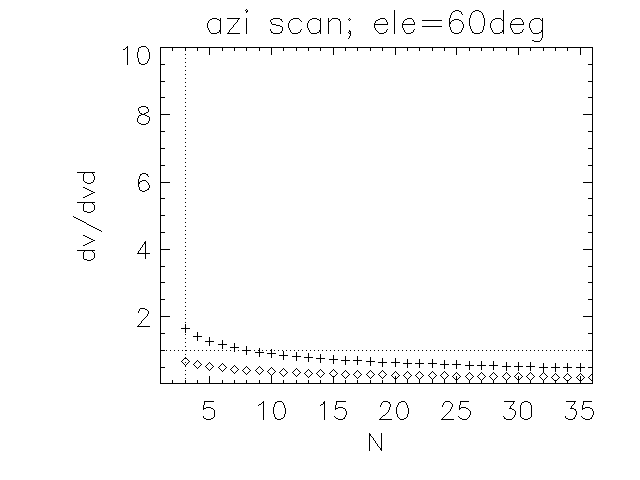full azi
+1 vertical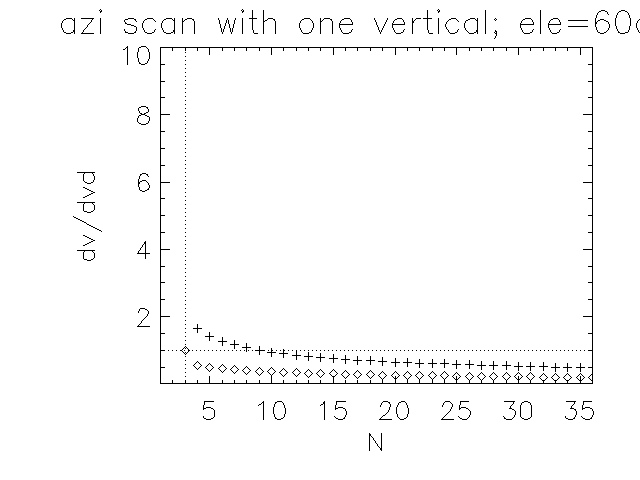full azi scan
+2 vertical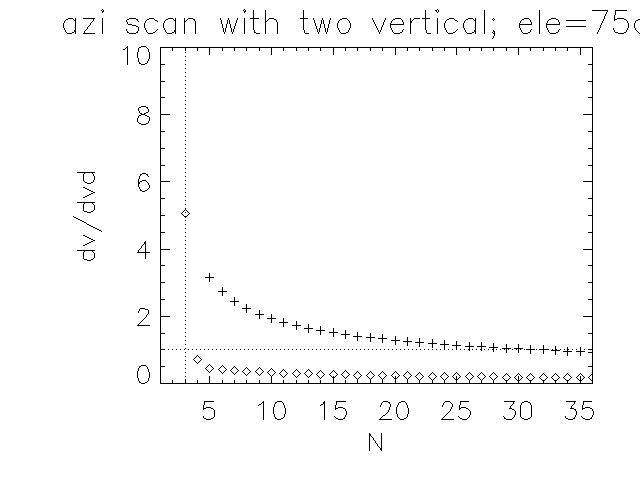sector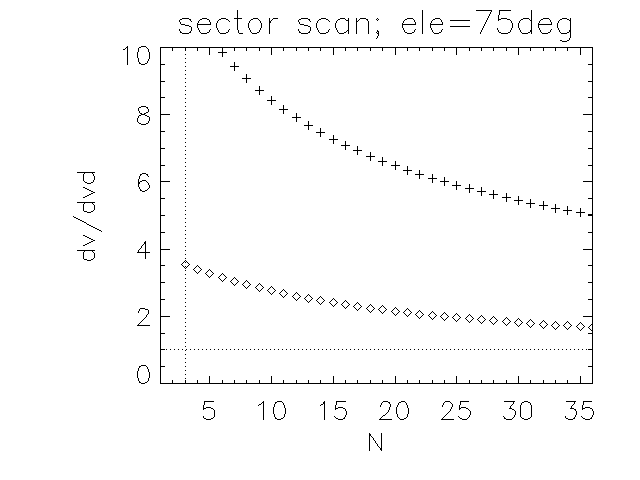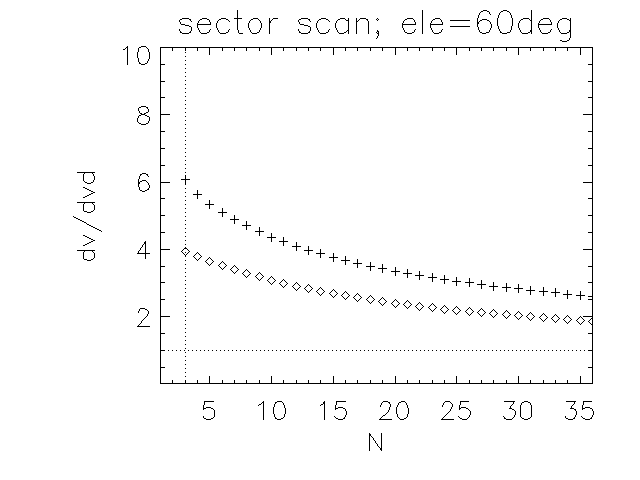sector
+1 vertical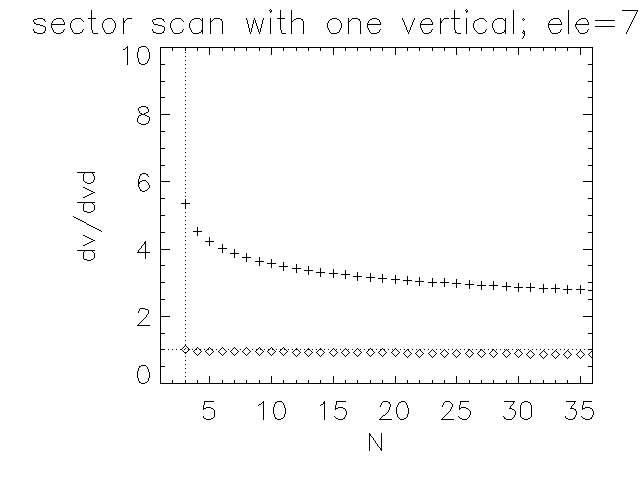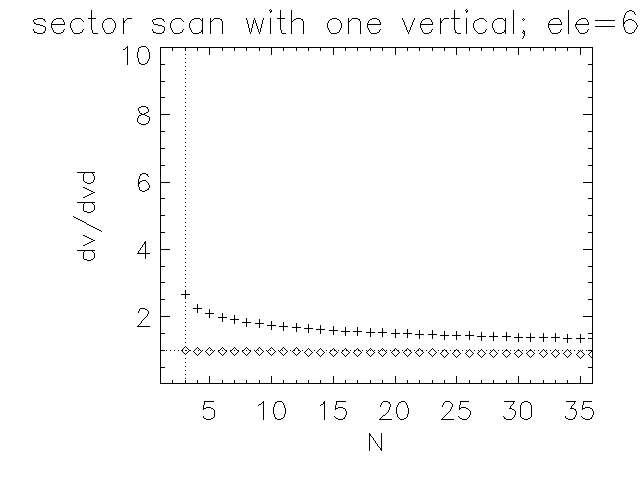sector
+2 vertical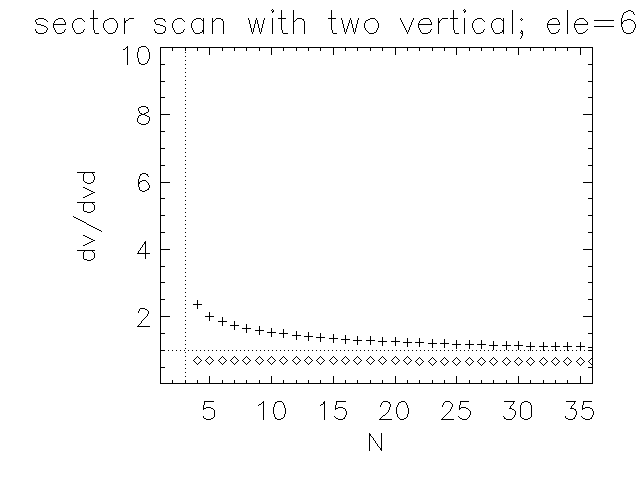halfhalf
+1 vertical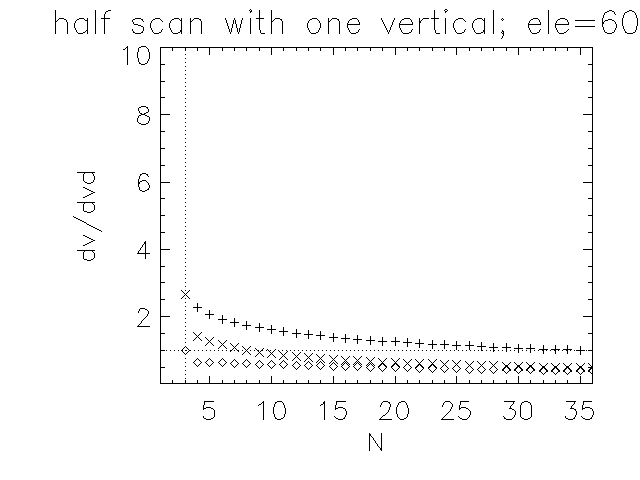half
+2 vertical